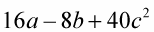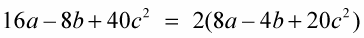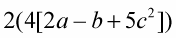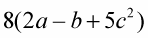##### Trigonometry Workbook For DummiesFactoring is the opposite of distributing. When distributing, you multiply a series of terms by a common factor. When factoring, you seek to find what a series of terms have in common and then take it away, dividing the common factor out from each term.

Think of each term as a numerator and then find the same denominator for each. By factoring out, the factor is put outside the parentheses or brackets and all the results of the divisions are left inside.

The proper way to factor an expression is to write the prime factorization of each of the numbers and look for the greatest common factor. A more practical and quicker way is to look for the largest factor that you can easily recognize. Factor it out and then see if the numbers within the parentheses need to be factored again. Repeat the division until the terms within the parentheses are relatively prime.

Example: Follow these steps to factor out the expression1. Determine a common factor.

A common factor is 2.

2. Divide each term by the common factor and write the results of the division in parentheses, with the factor out in front.3. Determine whether you can factor out any other terms.

The terms left in the parentheses are still too large. They all still a common factor of 4. Factoring out 4, you get: